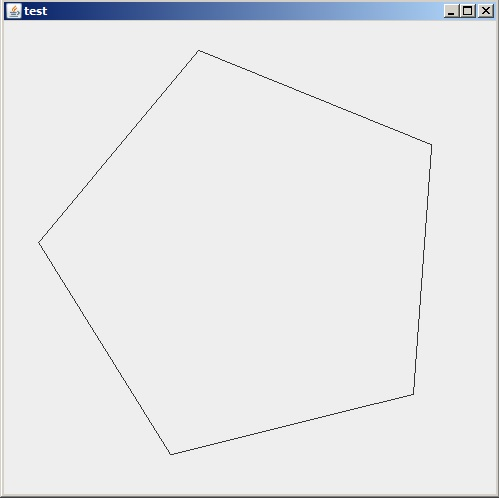Learn to Code
John F. Dumas

Why Learn Java?

► Unlike languages such as C or C++, java comes with everything you need to build gui programs right out-of-the-box.

Here's an example program that shows a rotating, 5-sided polygon. Observe that only about 80 lines of code are required to create this application. Here's the source and a screenshot of the running application (file: test.java):

• ```import java.awt.event.ActionListener;
import java.awt.event.ActionEvent;
import javax.swing.JPanel;
import javax.swing.JFrame;
import javax.swing.Timer;
import java.awt.Graphics;

public class test extends JPanel
{
public test()
{
mTimer = new Timer(
100, new ActionListener()
{
public void actionPerformed(ActionEvent e)
{
if(++mDegrees == 360) mDegrees = 0;
repaint();
}
}
);

mTimer.start();
}

public void paintComponent(Graphics g)
{
super.paintComponent(g);

int w = getWidth();
int h = getHeight();

int size = (int)(.45 * Math.min(w, h) + 0.5);

int xFirst = 0, yFirst = 0, xOld = 0, yOld = 0;

for(int i = 0, degrees = mDegrees; i < N_SIDES; i ++, degrees += STEP)
{

int x = (int)(w / 2 + size * Math.cos(radians) + 0.5);
int y = (int)(h / 2 + size * Math.sin(radians) + 0.5);

if(i == 0)
{
xFirst = x;
yFirst = y;
}
else
{
g.drawLine(x, y, xOld, yOld);

if(i + 1 == N_SIDES)
g.drawLine(x, y, xFirst, yFirst);
}

xOld = x;
yOld = y;
}
}

private Timer mTimer;
private int   mDegrees;

private static final int N_SIDES = 5;
private static final int STEP    = (360 / N_SIDES);

public static void main(String[] args)
{
JFrame j = new JFrame("test");

j.setDefaultCloseOperation(JFrame.EXIT_ON_CLOSE);
j.setSize(500, 500);

j.setVisible(true);
}
}
```

Results:Source Code

► Java has been around for more than 20 years and as a consequence there are many, many libraries, tools and packages already written to address common needs.

► Java is popular. As of July, 2021, the TIOBE Index of programming popularity was:

1. C
2. Java
3. Python
4. C++
5. C#

► Java is compiled down to bytecode class files and runs on the Java Virtual Machine. There are a number of other languages that also operate on the JVM and interacting with them from java is easy and straightforward.

► ONLINE RESOURCES

► ONLINE JAVA COMPILERS

► BOOKS

Back to Examples Index

© John F. Dumas | johnfdumas@gmail.com | main page | top of page